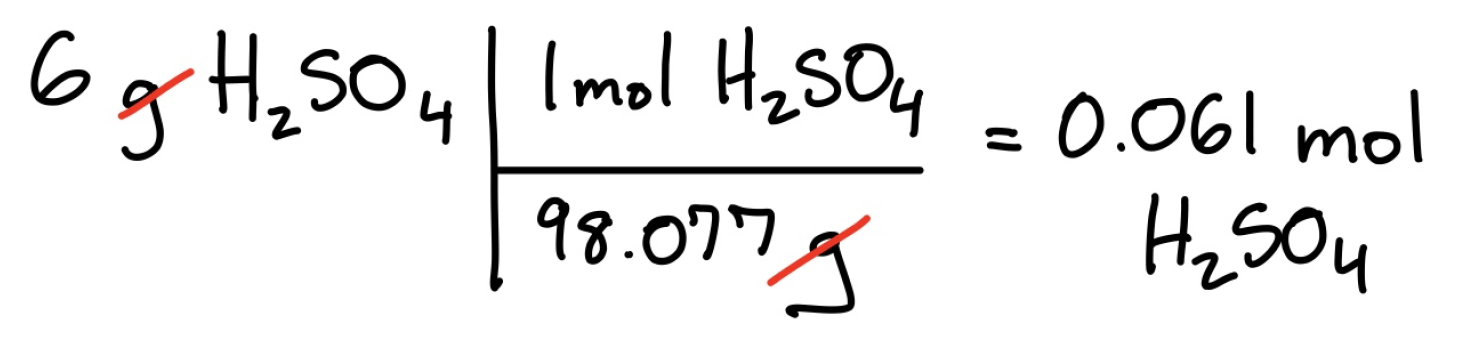Cyber Monday Sale: ALL MCAT Products & Admissions Services

MCAT Content / Stoichiometry / Molecular Weight

### Molecular Weight

Topic: Stoichiometry

The molecular weight of a total mass of atoms in a molecule, represented by grams per mole (g/mol).

The term molecular weight is most commonly seen to describe two things: The mass of a singular element and the mass of a substance with two or more elements. From the period table, we can directly see that the molecular weight of Hydrogen, H, is 1.008 g/mol.

However, when describing the molecular weight of a compound (a substance made of 2 or more elements), we must consider the molecular weight of all elements present. For example, if we wanted to determine the molecular weight of sulfuric acid, H2SO4, we could add the molecular weights of each element together:Note that each element is multiplied by the subscript, the small number to the right of the element, which represents the number of atoms of that element. Elements without subscripts are assumed to have one atom.

The molecular formula can be used with other SI for conversions, such as converting grams to mols. The conversion of grams of sulfuric acid, H2SO4, to moles.Practice Questions

Cell membranes and trafficking disorders

MCAT Official Prep (AAMC)

Chemistry Question Pack Passage 18 Question 100

Chemistry Question Pack Passage 19 Question 109

Key Points

• The molecular weight is represented by g/mol.

• The subscript of an element is multiplied by the molecular weight when adding up the molecular weights of individual elements in a compound; elements without a subscript following their elemental abbreviation can be assumed to have one atom.

Key Terms

compound: a substance made of two or more elements.

subscript:  number written below and immediately after an element that indicates the number of atoms of that element present.

Billing Information